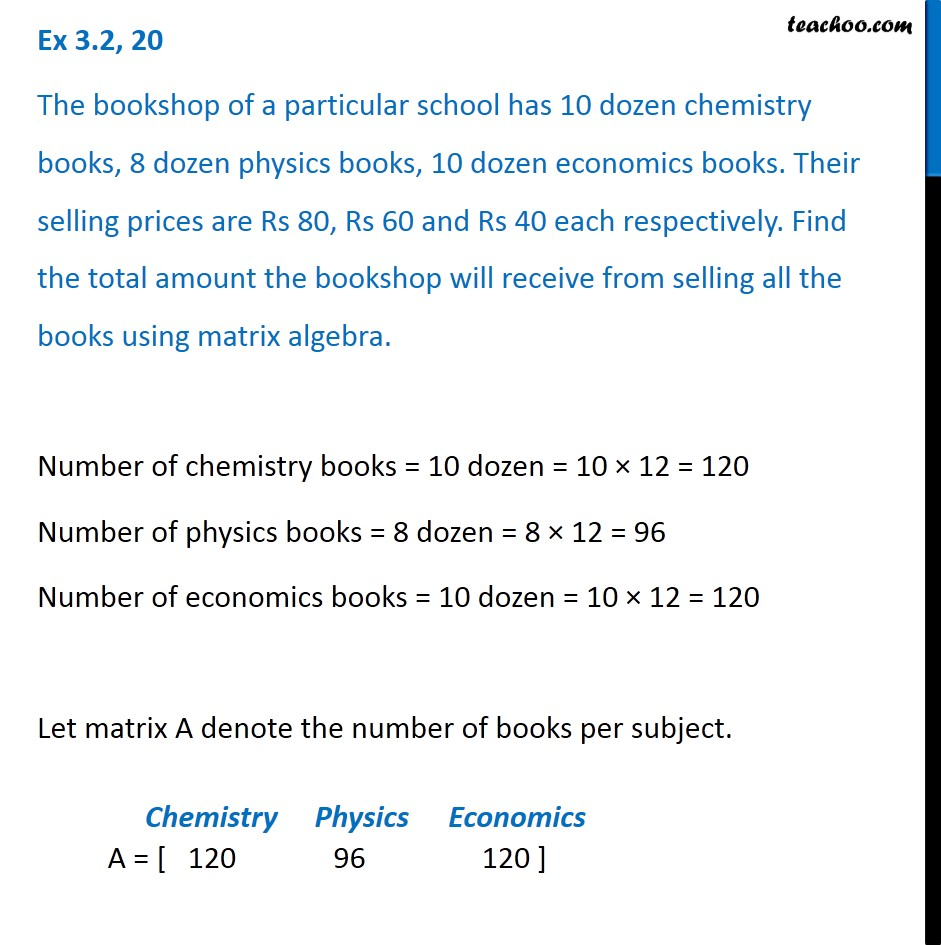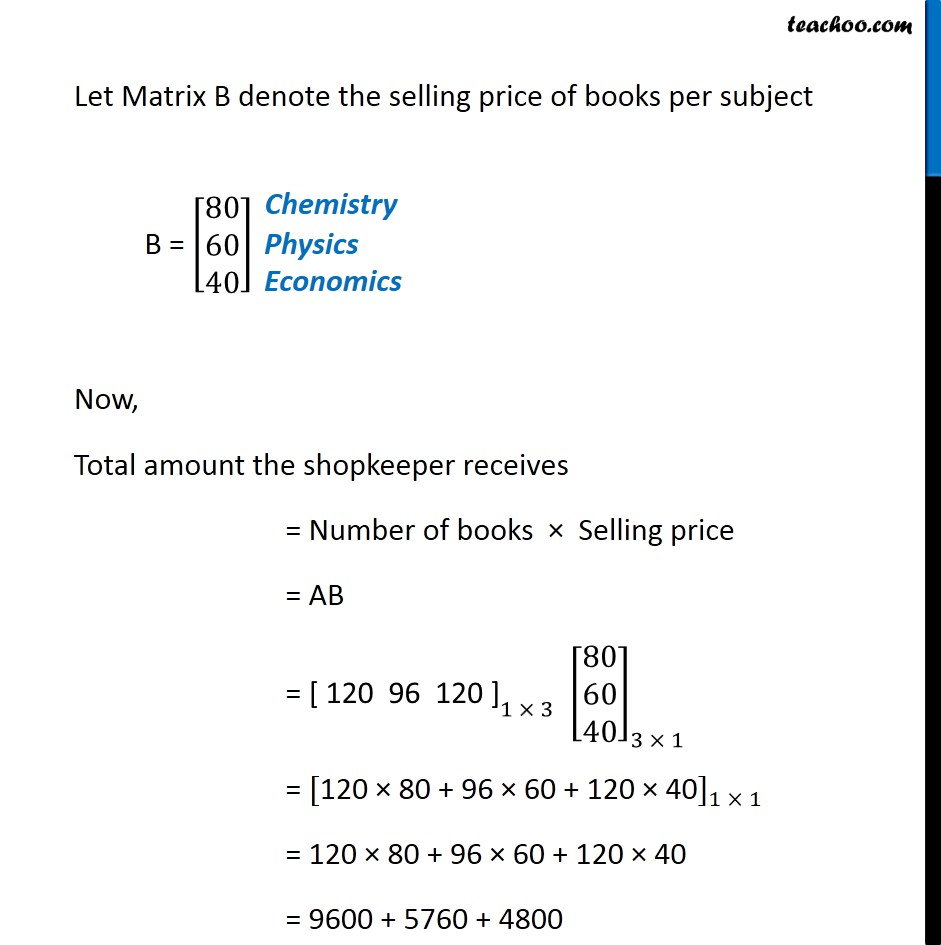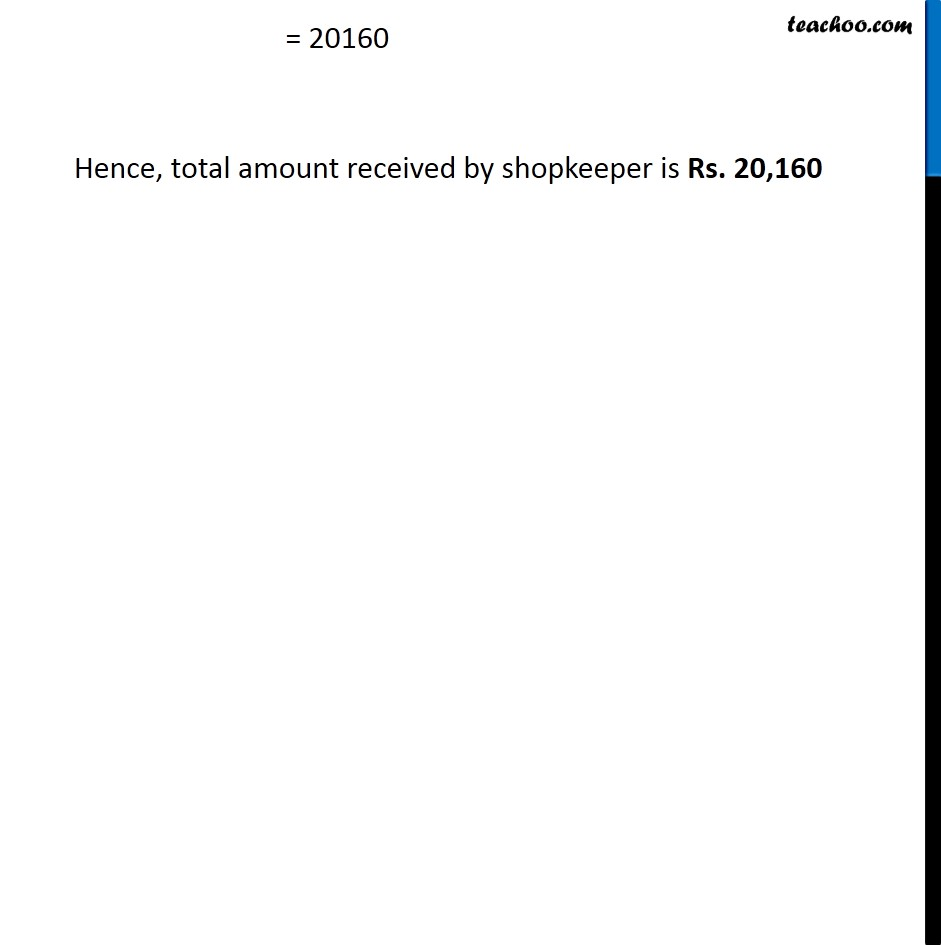1. Class 12
2. Important Questions for exams Class 12
3. Chapter 3 Class 12 Matrices

Transcript

Ex 3.2, 20 The bookshop of a particular school has 10 dozen chemistry books, 8 dozen physics books, 10 dozen economics books. Their selling prices are Rs 80, Rs 60 and Rs 40 each respectively. Find the total amount the bookshop will receive from selling all the books using matrix algebra. Number of chemistry books = 10 dozen = 10 × 12 = 120 Number of physics books = 8 dozen = 8 × 12 = 96 Number of economics books = 10 dozen = 10 × 12 = 120 Let matrix A denote the number of books per subject. A = [ 120 96 120 ] Let Matrix B denote the selling price of books per subject B = [█(80@60@40)] Now, Total amount the shopkeeper receives = Number of books × Selling price = AB = 〖"[ 120 96 120 ]" 〗_(1 × 3) [■8(80@60@40)]_(3 × 1) = 〖["120 × 80 + 96 × 60 + 120 × 40" ]〗_(1 × 1) = "120 × 80 + 96 × 60 + 120 × 40" = 9600 + 5760 + 4800 Chemistry = 20160 Hence, total amount received by shopkeeper is Rs. 20,160

Chapter 3 Class 12 Matrices

Class 12
Important Questions for exams Class 12